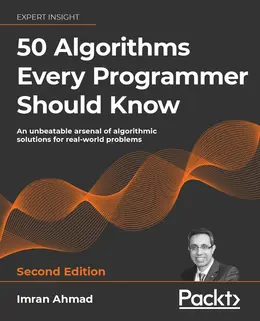# 50 Algorithms Every Programmer Should Know, Second Edition### eBook Details:

• Paperback: 497 pages
• Publisher: WOW! eBook (October 10, 2023)
• Language: English
• ISBN-10: 1803247762
• ISBN-13: 978-1803247762

### 50 Algorithms Every Programmer Should Know, 2nd Edition: Leverage algorithms to solve real world problems ranging from sorting and searching to modern algorithms in machine learning and cryptography

The ability to use algorithms to solve real-world problems is a must-have skill for any developer or programmer. This 50 Algorithms Every Programmer Should Know, Second Edition book will help you not only to develop the skills to select and use an algorithm to tackle problems in the real world by understanding how it works.

You’ll start with an introduction to algorithms and discover various algorithm design techniques, before exploring how to implement different types of algorithms, with the help of practical examples. As you advance, you’ll learn about linear programming, page ranking, and graphs, and even work with machine learning algorithms to understand the math and logic behind them.

Case studies will show you how to apply these algorithms optimally before you focus on deep learning algorithms and will learn about different types of deep learning models along with their practical use.

You will also learn about modern sequential models and their variants, algorithms, methodologies, and architectures that used to implement Large Language Models (LLMs) such as ChatGPT.

Finally, you’ll become well versed in techniques that enable parallel processing, giving you the ability to use these algorithms for compute-intensive tasks.

• Design algorithms for solving complex problems
• Become familiar with neural networks and deep learning techniques
• Explore existing data structures and algorithms found in Python libraries
• Implement graph algorithms for fraud detection using network analysis
• Use machine learning algorithms to process Twitter data in real time
• Create a recommendation engine that suggests relevant movies to subscribers
• Implement security using symmetric and asymmetric encryption on Google Cloud Platform

By the end of this 50 Algorithms Every Programmer Should Know, 2nd Edition programming book, you’ll have become adept at solving real-world computational problems by using a wide range of algorithms.